Ticker Tape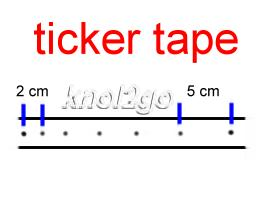The ticker tape can be used to study linear motion. The tape is usually attached to, and pulled by, a moving trolley. The analysis of the tape enables one to better understand the motion of the trolley. From the frequency of the ticker tape, one can get the time. From the lengths between the dots, the distance travelled by the trolley in the known time can be measured. Using the two quantities (distance and time), velocity can be calculated - and from it. acceleration can be derived.

For example, in the example above, with a frequency of 50Hz, the
time between two dots is
1/50Hz = 0.02s

The final velocity can be calculated as such

5cm/0.02s = 250cm/s

The initial
velocity can be calculated as such

2cm/0.02s =  100cm/s

The
time between the  final velocity and initial velocity is 5 X 0.02s = 0.1s

Thus, the
acceleration of the trolley can be calculated as

final velocity - initial velocity
time

= 250cm/s - 100cm/s
0.1s

= 1500cm/s2
= 1.5 m/s2

If this knol is helpful, please Like and Share it. Thanks.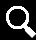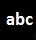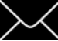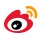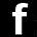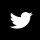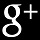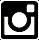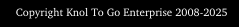Sila lihat di atas untuk pengiraan melibatkan pita detik.

Sekira knol ini memanfaatkan anda, sila tekan Like dan Kongsikannya. Terima kasih.